Function Repository Resource:

# PivotTable

A spreadsheet-like operation to collate data by values common to specified columns, summarizing corresponding values in a specified column

Contributed by: Kelvin Mischo
 ResourceFunction["PivotTable"][{{label1,label2,…},{i1,i2,…},{i3,i4,…}},binlabel,valuecolumn,func] collates values of valuecolumn common to binlabel and applies func to them. ResourceFunction["PivotTable"][{{label1,label2,…},{i1,i2,…},{i3,i4,…}}, binlabel1,binlabel2,valuelabel,func] collates values common to the two bin labels.

## Details and Options

The output contains as many rows as unique elements in binlabel or binlabel1.
The output contains two columns if one binlabel is specified, and three columns if a binlabel1 and binlabel2 are specified.
Common values for func are Total or Length, but any other function can be used.
The first argument can be a nested list or a variable storing an imported dataset as a nested list.

## Examples

### Basic Examples (2)

Get the Total of the "Second" column with a common name in the "First" column:

 In:=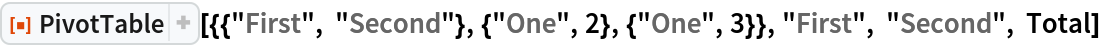Out=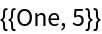Example dataset with labels in the first rows:

 In:=In:=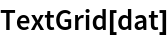Out=List all unique values in the column "City" and sum the corresponding numeric values in "Item 1" using Total:

 In:=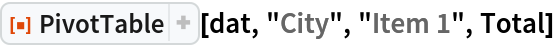Out=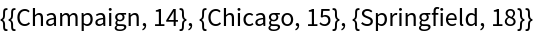Perform the same calculation, but format in a grid with TextGrid:

 In:=Out=List all unique values in the column "City" and sum the corresponding numeric values in "Item 2" using Total; use TextGrid in postfix form:

 In:=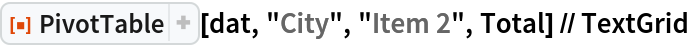Out=List all unique values common to both the "City" and "State" columns and sum the corresponding numeric values in "Item 1" using Total:

 In:=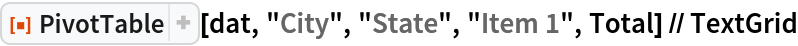Out=List all unique values common to both the "City" and "State" columns and sum the corresponding numeric values in "Item 2" using Total:

 In:=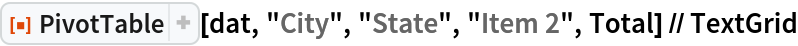Out=### Scope (6)

Example dataset with labels in the first rows:

 In:=List all unique values common to both the "City" and "State" columns and count the corresponding numeric values in "Item 2" using Length:

 In:=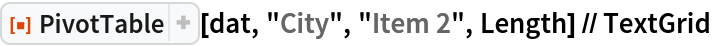Out=List all unique values common to both the "City" and "State" columns and count the corresponding numeric values in "Item 1" using Length:

 In:=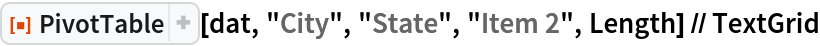Out=Perform the same calculation, with additional options for TextGrid to add a gray frame:

 In:=Out=Perform the same calculation, with different columns and Total to sum values in "Item 1":

 In:=Out=Although strings are commonly binned with pivot tables, numeric values can be used as well:

 In:=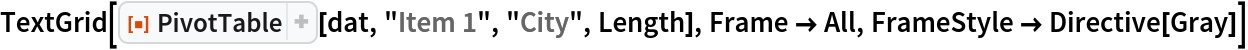Out=### Applications (2)

Example dataset with labels in the first rows:

 In:=Make a Table of pivot tables to compare values:

 In:=Out=Example dataset with labels in the first rows:

 In:=Perform a simple pivot table and export the resulting list:

 In:=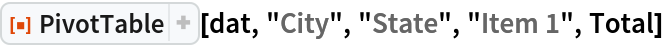Out=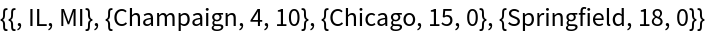In:=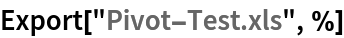Out=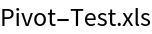### Neat Examples (2)

Example dataset with labels in the first rows:

 In:=Make a Manipulate with varying column values for a pivot table:

 In:=Out=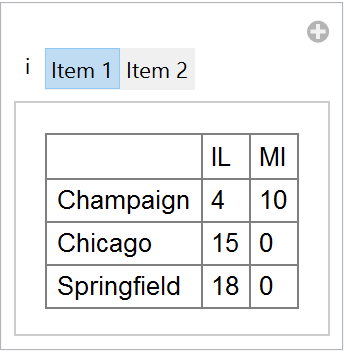Create a pivot table for a large dataset; first create a large test dataset:

 In:=In:=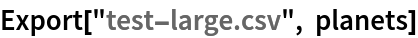Out=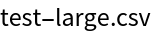Import the test dataset with 1.2 million rows and 4 columns:

 In:=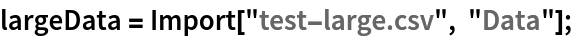In:=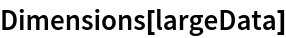Out=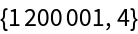Create a pivot table based on the large dataset:

 In:=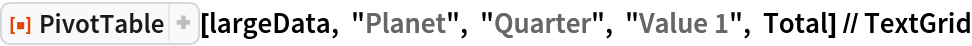Out=Kelvin Mischo

## Version History

• 1.0.0 – 12 August 2019